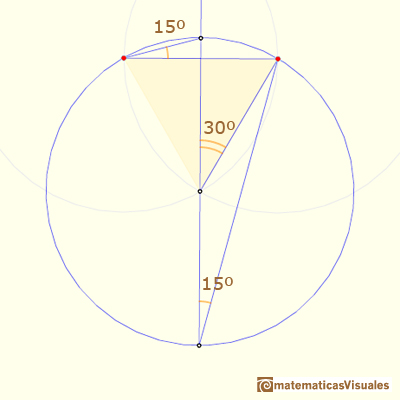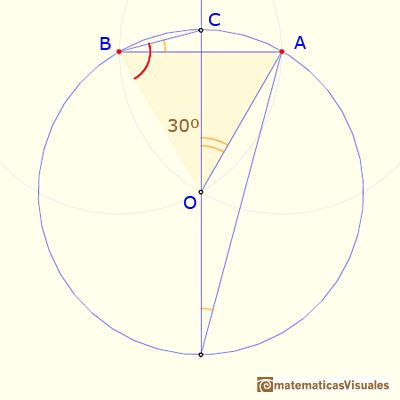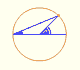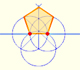Fifteen degrees angle

In this page we are going to see two ways to draw a 15º angle with a ruler and a 'rusty' compass (a compass of fixed opening). These are very simple constructions and there are, of course, many other ways to do the same (bisecting a 60º angle, subtracting 45º from 60º, ...).

They are basic examples of application of the properties of the angles central and inscribed in a circle (although, as we are going to see later, we can use more basic arguments involving some isosceles triangles).

The first construction is straightforward, and the result is very easy to see as a simple case of the property of angles central and inscribed in a circle:Another basic approach follows. In the next image, the triangle CBO is an isosceles triangle, then:And we can calculate the angle:

Now we are going to see a second construction of a 15º angle:

Again it is an example of the property of the angles central and inscribed in a circle:In the next image, we can see that the triangle CB0 is an isosceles triangle, then:To finish, we can calculate the angle:Interactive 'Mostation' of the property of central and inscribed angles in a circle. Case II: When one chord that forms the inscribed angle is a diameter.Interactive 'Mostation' of the property of central and inscribed angles in a circle. The general case is proved.Each point in the circle circunscribed to a triangle give us a line (Wallace-Simson line)Interactive demonstration of the Wallace-Simson line.You can draw a regular pentagon given one of its sides constructing the golden ratio with ruler and compass.In his book 'Underweysung der Messung' Durer draw a non-regular pentagon with ruler and a fixed compass. It is a simple construction and a very good approximation of a regular pentagon.The diagonal of a regular pentagon are in golden ratio to its sides and the point of intersection of two diagonals of a regular pentagon are said to divide each other in the golden ratio or 'in extreme and mean ratio'.